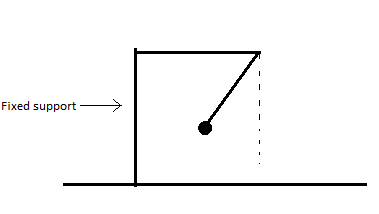# The pendulum effectAn experiment based on pendulum is being done on ground, as shown in the above diagram

A Pendulum with a bob of mass $1Kg$ and negligible size is attached to a fixed support which is fixed to the ground and is released at an angle $\theta$ with the vertical. When the bob reaches the lower most point a sophisticated machine measures it's velocity as $1.00 m/sec$Now the same experiment is being done with the fixed support being fixed on a cart which is itself is placed on a very smooth ground(cart is free to move and currently at rest) and the pendulum is attached to the fixed support exactly as in the first experiment.

Also this time the measuring machine is placed on the cart itself(measuring machine has negligible mass). Again pendulum is released from the same angle $\theta$ with the vertical.

This time that machine measures the velocity of the bob when it was at it's lowest position as $1.01 m/s$. Find the mass of the cart in $Kg$

Details and Assumptions

1)Assume the mass of the fixed support to be neglible.

×